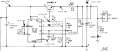# Question about uA723 with external pass transistor with foldback current limiting

#### szabikka

Joined Sep 3, 2014
113
Hello Forum Members,

I have come across this circuit schematic in the service guide of an old arcade machine:I started to do some calculations but I'm not sure if I made a mistake. I tried to calculate where the current limiting turns on, i.e. the knee-current in case of foldback limiting. This was my line of thinking: The 723 will do whatever it can to keep the output voltage of the circuit at 12.1 V; Under the circumstances presented here (the voltages included in the schematic) the current across R14 is (12.3-12.1)/0,27 = 0.74A. As we can see the voltage at the CL pin (base of the current limiting internal transistor) is lower than the voltage on the CS pin (emitter of said transistor), hence the current limiting doesn't turn on). To turn the current limiting on, the voltage of CL pin has to reach a value that is 0.7V higher than the voltage on the CS pin. Since the voltage at the CS pin is held at 12.1V, the voltage on the CL pin has to be around 12.8V for current limiting to start. If we take into consideration the voltage divider presented by R11 and R13, a voltage of 12.8V is present on the CL pin when the voltage of the VO pin is about 14V. If 14V is present on the base of the external pass transistor, then its emitter voltage will be 13.4V. In this case the current through R14 is (13.4-12.1)/0.27 = 4.81A. So this should be the knee-current. Problem is that the power of R14 in this case is (13.4-12.1)x4,81 = 6.25W, more than double of its power rating, so it will burn out far before current limiting occurs. Is something wrong with my calculation or thinking?

One more thing, I tried to use the formula presented on page 5 of the uA723 datasheet for foldback current limiting. And if I put the values of the circuit into the Iknee formula, I get an even higher knee-current, 6.74A. Am I completely on the wrong track?

I'm attaching the datasheet for the uA723 regulator and the service guide of the arcade machine where the circuit schematic comes from.

Thank you for the help in advance!

#### Attachments

• 557.9 KB Views: 9
• 2.8 MB Views: 6

#### szabikka

Joined Sep 3, 2014
113
Thanks Eric! Looks like none of my calculations are in line with the simulation. I don't think the transistor type would have such a big impact on the results. Guess I will have to construct the circuit (with what I have) and do some measurements.

#### szabikka

Joined Sep 3, 2014
113
Eric, I can see that you've been using the datasheet from National Semiconductor. In that datasheet (that I will attach, just so other can see it too) in the typical performance characteristics there is a graph that shows the current sense voltage against the junction temperature. From those curves it's obvious that the current sense voltage is between 0.5V and 0.8V, depending on the sense resistor and the temperature. I did the calculation - using the formula for current foldback limiting from the datasheet - for the schematic that you have used in the spice simulation and I got a knee-current of 110 mA, which correlates with the red trace (current through R7) in your simulation. For sense voltage I used 0.65V, so it seems to be the good one. A 1.1V sense voltage would suggest a darlington transistor for current limiting but it's not the case for lm723 nor uA723.

Looks like the formula for foldback current limiting works fine as long as there is no external pass transistor included or maybe the people designing the original circuit I posted, used a resistor with a too low power rating.

#### Attachments

• 213.6 KB Views: 3
•ericgibbs

#### szabikka

Joined Sep 3, 2014
113
hi @szabikka
Problem solved,
The equation in the d/s applies the circuit in the d/s, your circuit is incorrect [ could be a drawing error]
Compare circuits and Cur/Lim result.
R2 connections
E
View attachment 296534
Wow, thank you for comparing the two circuits Eric!
From these it's obvious that both my calculations were somewhat "correct". On the left you used the schematic from the original post. For that I calculated a knee current of 4.81A and according to your simulation it's around 4.0A. The second schematic correlates with the datasheet, but includes an external pass transistor. For this I calculated a knee current of 6,71A and according to the sim it's about 6.6A.

Looks like both circuits work, but the first one has a lower knee current and sharp current and voltage drop after current limiting kicks in, the second one behaves more like a textbook foldback current limiting with a sloped voltage and current drop.

•ericgibbs